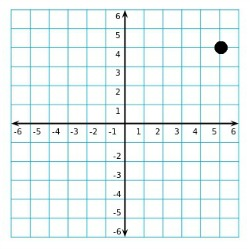# Coordinate Graph: Definition & Examples

Instructions:

Choose an answer and hit 'next'. You will receive your score and answers at the end.

question 1 of 3

### What is the ordered pair that represents the position of the point shown on the coordinate graph?Create Your Account To Take This Quiz

As a member, you'll also get unlimited access to over 75,000 lessons in math, English, science, history, and more. Plus, get practice tests, quizzes, and personalized coaching to help you succeed.

Try it risk-free for 30 days. Cancel anytime.

### 2. Consider the ordered pair (7, 14). What number does this ordered pair line up with on the y-axis when plotted on a coordinate graph?

Create your account to access this entire worksheet
A Premium account gives you access to all lesson, practice exams, quizzes & worksheets
Quizzes, practice exams & worksheets
Certificate of Completion
Create an account to get started

You're going to be assessed predominantly on your grasp of ordered pairs and properties of coordinate graphs with this quiz/worksheet.

## Quiz & Worksheet Goals

Your comprehension of the following subjects will be tested:

• The relationship between the y and x-coordinate
• Graphing ordered pairs
• Identifying ordered pairs on a graph
• Qualities of coordinate graphs

## Skills Practiced

• Distinguishing differences - compare and contrast topics from the lesson, such as the y-coordinate and the x-coordinate
• Information recall - access the knowledge you've gained regarding graphing points
• Critical thinking - apply relevant concepts to examine information about the history of graphing in a different light

Discover more about graphing with our corresponding lesson, Coordinate Graph: Definition & Examples. This lesson with advance your understanding of:

• Rene Descartes' contribution to graphing
• Components of a coordinate graph
• Plotting ordered pairs on a graph
Final ExamACT Prep: Tutoring Solution
Status: Not Started
Chapter ExamACT Math - Coordinate Geometry: Tutoring Solution
Status: Not Started

Support